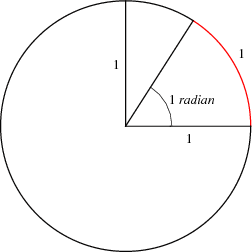Related Articles
ISRO | ISRO CS 2014 | Question 9
• Last Updated : 11 May, 2018

Let be the radius of the circle. What is the angle subtended by an arc of length at the center of the circle?
(A) 1 degree
(B) 1 radian
(C) 90 degrees
(D) π radians

Answer: (B)

Explanation: Radian describes the plane angle subtended by a circular arc as the length of the arc divided by the radius of the arc. One radian is the angle subtended at the center of a circle by an arc that is equal in length to the radius of the circle.Note that 1 radian = 57.2958 degree.

So, option (B) is correct.

Quiz of this Question

Attention reader! Don’t stop learning now. Get hold of all the important DSA concepts with the DSA Self Paced Course at a student-friendly price and become industry ready.

My Personal Notes arrow_drop_up